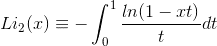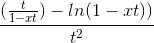# Expanding Integrand into Power Function

soothsayer

## Homework Statement

The dilogarithm Li2(x) is a special function defined by the following integralExpand the integrand in a power series and integrate term by term, thereby deriving the power series expansion for Li2 about x=0

## Homework Equations

f(x)= f(0) + xf'(0) + (x2/2)f''(0) + (x3/3!)f'''(0)
formula for Maclaurin series.

## The Attempt at a Solution

So, as far as I can discern, I take the integrand of the dilogarithmic function, and treat it as a function itself by expanding it into the Taylor power series around x=0 (Maclaurin series). This means that I will be differentiating with respect to t, correct? Since I will later have to integrate term-by-term over t, so I treat the integrand as a function of t. The first two terms are pretty simple, but I have trouble getting past that. Here's what I have so far:
f(0) = ln(1-0*t)/t = ln(1) = 0
f'(t) =f'(0) = 1/(1-t)

I can't differentiate past this. Actually, I tried and came up with 1/2(1-t) which was nice because it followed a pattern, but when I integrated term by term, I ended up with natural logs of 0 which totally ruined the calculations.

I'm also not sure I understand the concept of expanding this sort of function into Taylor Series, the original function is of x, but the integral is over dt, do I expand using t as the variable or x? I know I'm suppose to approximate the function at x=0. Any insight on this problem would be great! I feel like I'm probably just differentiating poorly.

## Answers and Replies

spamiam
There's a much easier way to find the power series representation for the log term. Try using
$$\ln(1-u) = \int \frac{-1}{1-u} \, dt$$

and then expanding $\frac{1}{1-u}$ as a geometric series.

In this definition, t is a dummy variable--it disappears once you perform the integration. However, the integrand is a function of both x and t, so inside the integral you need to expand in both x and t. (I.e., set u = xt).

lswtech
Why not start with figuring out ln(1-xt) in something of t and divide it :)

HACR
There's a much easier way to find the power series representation for the log term. Try using
$$\ln(1-u) = \int \frac{-1}{1-u} \, dt$$

and then expanding $\frac{1}{1-u}$ as a geometric series.

In this definition, t is a dummy variable--it disappears once you perform the integration. However, the integrand is a function of both x and t, so inside the integral you need to expand in both x and t. (I.e., set u = xt).

The integral ends up being [tex]\int_0^1 \frac {(x/2+x^2/3+x^3/4+x^4/5+...)}{t}dt But still the t terms would be integrated to give ln(t), but ln(t) actually has a singularity or infinite discontinuity at t=0.

spamiam
Ah okay, perhaps this is more subtle than I first thought. I did a little "Feynman integration" to solve the problem. That is, I first calculated the Taylor series for the derivative ##\text{Li}_2'(x)## by exchanging the integral and derivative operators
$$\text{Li}_2'(x) = - \frac{d}{dx} \int_0^1 \frac{\log(1 - xt)}{t} \, dt = - \int_0^1 \frac{\partial}{\partial x} \frac{\log(1 - xt)}{t} \, dt$$
which is hopefully justified by the dominated convergence theorem. That should eliminate the problem with log(t) you pointed out, HACR. Then you can easily integrate that series to obtain the Taylor series for ##\text{Li}_2(x)##. Thanks for pointing out my mistake!

HACR
Right you used the leibnitz rule. Anytime.